Past Year Questions: Tacheometric, Curve, Hydrographic and Plane Table Surveying & Theory of Errors

# Past Year Questions: Tacheometric, Curve, Hydrographic and Plane Table Surveying & Theory of Errors Notes | Study Topic wise GATE Past Year Papers for Civil Engineering - Civil Engineering (CE)

## Document Description: Past Year Questions: Tacheometric, Curve, Hydrographic and Plane Table Surveying & Theory of Errors for Civil Engineering (CE) 2022 is part of Civil Question Bank for Topic wise GATE Past Year Papers for Civil Engineering preparation. The notes and questions for Past Year Questions: Tacheometric, Curve, Hydrographic and Plane Table Surveying & Theory of Errors have been prepared according to the Civil Engineering (CE) exam syllabus. Information about Past Year Questions: Tacheometric, Curve, Hydrographic and Plane Table Surveying & Theory of Errors covers topics like and Past Year Questions: Tacheometric, Curve, Hydrographic and Plane Table Surveying & Theory of Errors Example, for Civil Engineering (CE) 2022 Exam. Find important definitions, questions, notes, meanings, examples, exercises and tests below for Past Year Questions: Tacheometric, Curve, Hydrographic and Plane Table Surveying & Theory of Errors.

Introduction of Past Year Questions: Tacheometric, Curve, Hydrographic and Plane Table Surveying & Theory of Errors in English is available as part of our Topic wise GATE Past Year Papers for Civil Engineering for Civil Engineering (CE) & Past Year Questions: Tacheometric, Curve, Hydrographic and Plane Table Surveying & Theory of Errors in Hindi for Topic wise GATE Past Year Papers for Civil Engineering course. Download more important topics related with Civil Question Bank, notes, lectures and mock test series for Civil Engineering (CE) Exam by signing up for free. Civil Engineering (CE): Past Year Questions: Tacheometric, Curve, Hydrographic and Plane Table Surveying & Theory of Errors Notes | Study Topic wise GATE Past Year Papers for Civil Engineering - Civil Engineering (CE)
 1 Crore+ students have signed up on EduRev. Have you?

Question 1: The method of orientation used, when the plane table occupies a position not yet located on the map, is called as    [2017 : 1 Mark, Set-II]
(a) traversing
(c) levelling
(d) resection

Solution: 1. Traversing: 'Traverse’ means to pass across. Traversing refer to the framework of series of lines forming an open or closed polygon.
2. Radiation: It is a method of locating a point by drawing a radial line from the plane table station to the station under consideration.
3. Levelling: It is the method of determining the difference of elevations or levels of different points on the earth’s surface.
4. Resection: It is the method of locating a station occupied by the plane table when the position of that station has not been plotted earlier on the sheet when the plane table occupied other station

Question 2: The VPI (vertical point of intersection) is 100 m away (when measured the horizontal) from the VPC (vertical point of curvature). If the vertical curve parabolic, the length of the curve (in meters and measured along the horizontal) is ________.    [2017 :1 Mark, Set-II]
Solution: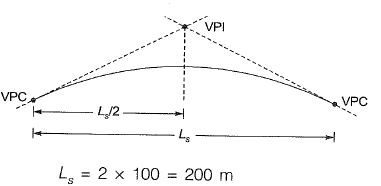Question 3: A circular curve of radius R connects two straights with a deflection angle of 60°. The tangent length is    [2016 : 1 Mark, Set-II]
(a) 0.577 R
(b) 1.155 R
(c) 1.732 R
(d) 3.464 R
Solution: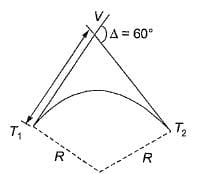Tangent length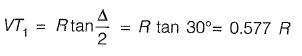Question 4: In a survey work, three independent angles, X, Y and Z were observed with weights Wx, WY, Wz, respectively. The weight of the sum of angles X, Y and Z is given by    [2015 : 2 Marks, Set-T]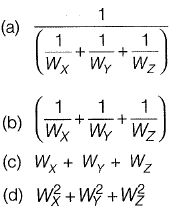Solution: Various laws of weight
Let there be quantities A and B with weight Wa and Wb respectively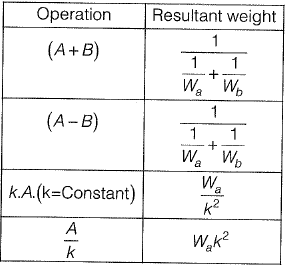Question 5: The chainage of the intersection point of two straights is 1585.60 m and the angle of intersection is 140°. If the radius of a circular curve is 600.00 m, the tangent distance (in m) and length of the curve (in m), respectively are    [2014 : 2 Marks, Set-II]
(a) 418.88 and 1466.08
(b) 218.38 and 1648.49
(c) 218.88 and 418.82
(d) 418.88 and 218.38
Solution: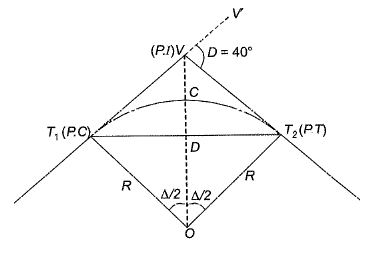Δ = 180° - 140° = 40°
Length of the curve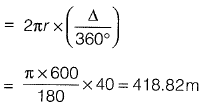Tangent distance (7) is the distance between
P-C to P.I (also the distance from P.I to P. T)
⇒ T = T1V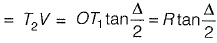= 600 tan 20° = 218.88 m

Question 5: A tacheometer was placed at point P to estimate the horizontal distances PQ and PR. The corresponding stadia intercepts with the telescope kept horizontal, are 0.320 m and 0.210 m, respectively. The ∠QPR is measured to be 61° 30' 30". If the stadia multiplication constant = 100 and stadia addition constant = 0.10 m, the horizontal distance (in m) between the points Q and R is ______.     [2014 : 2 Marks, Set-II]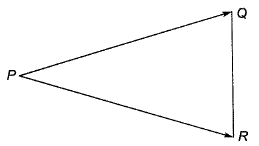PQ = ks + C
= 100(0.32) + 0.1 = 32.1 m
PR = ks + C
= 100(0.21) + 0.1 = 21.1 m
Applying the cosine rule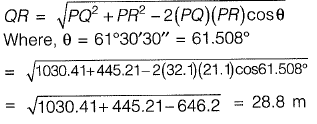The document Past Year Questions: Tacheometric, Curve, Hydrographic and Plane Table Surveying & Theory of Errors Notes | Study Topic wise GATE Past Year Papers for Civil Engineering - Civil Engineering (CE) is a part of the Civil Engineering (CE) Course Topic wise GATE Past Year Papers for Civil Engineering.
All you need of Civil Engineering (CE) at this link: Civil Engineering (CE)

## Topic wise GATE Past Year Papers for Civil Engineering

67 docs
 Use Code STAYHOME200 and get INR 200 additional OFF

## Topic wise GATE Past Year Papers for Civil Engineering

67 docs

Track your progress, build streaks, highlight & save important lessons and more!

,

,

,

,

,

,

,

,

,

,

,

,

,

,

,

,

,

,

,

,

,

,

,

,

,

,

,

;